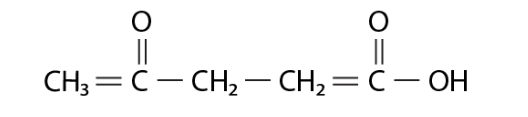# Find the equation of a line which is equidistant from the lines`
Question:

Find the equation of a line which is equidistant from the lines x = - 2 and x = 6

Solution:

For the equation of line equidistant from both lines, we will find point through which line passes and is equidistant from both line.

As any point lying on $x=-2$ line is $(-2,0)$ and on $x=6$ is $(6,0)$, so mid - point is

$(\mathrm{x}, \mathrm{y})=\left(\frac{-2+6}{2}, \frac{0+0}{2}\right)$

$(x, y)=(2,0)$So, equation of line is x = 2.